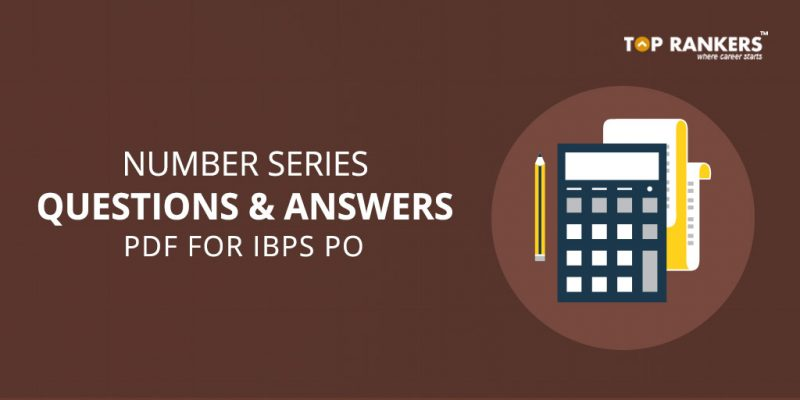Institute of Banking Personnel Selection conducts the IBPS PO Exam every year to recruit for Probationary Officers in the Public sector banks. This post will help in preparing for Number Series Questions asked in the IBPS Exam. The direct link to Download Free IBPS PO Number series Questions and Answer PDF has been mentioned below.

This Quantitative Aptitude PDF is basically a study-guide which will help in preparing questions on “Number Series/Quantitative Aptitude” and in turn help in getting good grades for the exam.

## IBPS PO Number series Questions and Answer

• Go through the IBPS PO Syllabus covers:- English LanguageLogical Reasoning, Quantitative Aptitude and Computer Aptitude
• Number Series is considered to be a challenging topic in the IBPS PO Exam.
• Many questions asked in the IBPS PO Quantitative Aptitude section are from number series. Along with the right preparation, the number series section can be very scoring.
• Go through the IBPS PO Preparation Tips to know about the right strategies, tips and study material for exam preparation.

### What is the Number Series?

Number series is a set of numbers in a certain sequence, where some numbers are mistakenly put into the series of numbers and some number is missing in that series. The candidate is expected to enter the correct number in the appropriate place.

1. Quantitative Aptitude section can be challenging in the IBPS PO Exam.
2. You can ace this section by practising regularly.
3. Number Series is one topic that can get scoring if you master the basics.• All Questions given in this PDF file are followed by 5 options, there is only 1 right option in each question.
• Attempt all questions in the IBPS PO Number series Questions and Answer PDF
• Once you have answered all questions you can view the answer key given at the end of the PDF file.

### Tips and Tricks for IBPS PO Number series Questions

• Number series questions are mostly numbered in the series are connected to each other. When you identify the connection, solving the question becomes easier for you.
• Identify the pattern asked in the question. Once you identify the pattern, solving the questions would be much easier.
• Once you have identified the pattern, apply it to the number before/ after the missing number in the series to get the answer.

### Common Patterns in Number Series

The common patterns asked in number series topic are-

1. Prime numbers
2. Squares/ cubes
3. Pattern in differences
4. Pattern in alternate numbers
5. Geometric Series
6. Odd one out
8. Complex Series
9. Using 2 or more arithmetic functions
10. Cube roots and Square roots
11. Alternate Primes
12. Third Number as the sum of the preceding 2 numbers1.suresh chandra yadav says:
2.suresh chandra yadav says: